Organic Chemistry : Help with Oxidation-Reduction Reactions

Example Questions

Example Question #1 : Help With Oxidation Reduction Reactions

Which of the following is not capable of oxidizing a secondary alcohol to a ketone?

Lithium aluminum hydride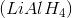All of these answers can oxidize secondary alcohols to ketones

Pyridinium chlorochromate (PCC)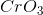,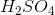, acetone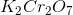Lithium aluminum hydrideExplanation:

Lithium aluminum hydride is correct because it is a reducing agent, and is therefore not capable of oxidizing secondary alcohols. Instead, LAH could be used to perform the reverse reaction, reducing a ketone to an alcohol. The other answer choices are oxidizing agents.

Example Question #2 : Help With Oxidation Reduction Reactions

In the following equation, which element is reduced?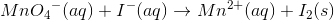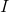Manganese, iodine, and oxygen are all oxidized.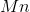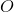Explanation:

In an oxidation-reduction (redox) reaction, reduction and oxidation both occur. Thus, not all of the elements can be oxidized, and not all of them can be reduced. In this equation, the oxidation number of oxygen is. Multiplying that by the number of oxygen atoms (), the overall charge on oxygen is.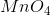has an overallcharge, so the oxidation number onmust combine withto form. Thus, the oxidation number ofat the beginning of the reaction is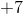. The iodine at the beginning of the reaction has an oxidation number of, as seen by the negative superscript.

At the end of the reaction,has an oxidation number of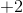, as seen by the positive superscript. Iodine has an oxidation number of(there is no charge on the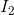).

Thus,went from having an oxidation number ofto one of. Iodine went from having an oxidation number ofto one of. Oxidation occurs when electrons are lost (the number becomes more positive), and reduction occurs when electrons are gained (the number becomes more negative). Because the oxidation number of iodine became more positive, iodine was oxidized. Because the oxidation number of manganese became more negative (less positive), manganese was reduced.

All Organic Chemistry Resources